# Measurement of finite-frequency current statistics in a single-electron transistor

## Abstract

Electron transport in nanoscale structures is strongly influenced by the Coulomb interaction that gives rise to correlations in the stream of charges and leaves clear fingerprints in the fluctuations of the electrical current. A complete understanding of the underlying physical processes requires measurements of the electrical fluctuations on all time and frequency scales, but experiments have so far been restricted to fixed frequency ranges, as broadband detection of current fluctuations is an inherently difficult experimental procedure. Here we demonstrate that the electrical fluctuations in a single-electron transistor can be accurately measured on all relevant frequencies using a nearby quantum point contact for on-chip real-time detection of the current pulses in the single-electron device. We have directly measured the frequency-dependent current statistics and, hereby, fully characterized the fundamental tunnelling processes in the single-electron transistor. Our experiment paves the way for future investigations of interaction and coherence-induced correlation effects in quantum transport.

## Introduction

The electrical fluctuations in a nanoscale conductor reveal a wealth of information about the physical processes inside the device compared with what is available from a conductance measurement alone1,2,3. Zero-frequency noise measurements are now routinely performed using standard techniques, but, more recently, the detection of higher order current correlation functions, or cumulants, has attracted considerable attention in experimental4,5,6,7,8,9,10,11,12,13,14,15,16,17,18,19 and theoretical20,21,22 studies of charge transport in man-made sub-micron structures. Measurements have, for example, been encouraged by the intriguing connections between current fluctuations and entanglement entropy in solid-state systems23,24 as well as by the possibility to test fluctuation theorems25,26,27 at the nanoscale that are now a topic at the forefront of non-equilibrium statistical physics.

Experiments on current fluctuations have mainly focused on zero-frequency current correlations4,5,6,7,8,9,10,11,12,13,14,15,16 whereas measurements of finite-frequency cumulants of the current have remained an outstanding experimental challenge. However, noise detection in a restricted frequency band gives only partial information about correlations and measurements that cover the full frequency range are necessary to access all relevant timescales that characterize the transport process. In a zero-frequency measurement, correlation effects are integrated over a long period of time and important information about characteristic timescales are lost. To observe the dynamical features of the correlations, finite-frequency measurements are required2,3,28,29,30,31,32,33,34. It has even been shown theoretically that the frequency-dependent third cumulant (the skewness) of the current may contain further information about correlations and internal timescales of a system compared with the finite-frequency noise alone, for instance in chaotic cavities29 and diffusive conductors30.

Figure 1a–c shows a typical time trace of the currents in our nanoscale single-electron transistor (SET) consisting of a quantum dot (QD) coupled through tunnelling barriers to source and drain electrodes. The QD is operated close to a charge degeneracy point in the Coulomb blockade regime, where only a single extra electron at a time can enter from the source electrode and leave through the drain electrode. The applied voltage bias eV is larger than the electronic temperature kBT which prevents electrons from tunnelling in the opposite direction of the mean current. While a stream of electrons is driven through the QD, a separate current is passed through the nearby quantum point contact (QPC) whose conductance is highly sensitive to the presence of extra electrons (Δq=0,1) on the QD35,36. By monitoring the switches of the current through the QPC, we can thus detect, in real-time, single electrons tunnelling through the left (L) and right (R) barriers and thereby determine the corresponding pulse currents IL(t) and IR(t). The time trace of the currents illustrates the electrical fluctuations of interest here.

We now analyse the current fluctuations obtained from data measured over 24 h during which 300 million electrons passed through the SET. Our high-quality measurements allow us to develop a complete picture of the noise properties of the SET, which not only focuses on the zero-frequency components of the fluctuations, but also contains the full frequency-resolved information about the noise and higher order correlation functions. From the measured finite-frequency noise spectrum, we extract the correlation time of the current fluctuations. The noise spectrum, however, is a one-frequency quantity only which does not reflect correlations between different spectral components of the current. To observe such correlations, we employ bispectral analysis and consider the finite-frequency skewness (or bispectrum) of the current. The skewness shows that the current fluctuations are non-gaussian on all relevant time and frequency scales owing to the non-equilibrium conditions imposed by the applied voltage bias. Our measurements are supported by model calculations that are in excellent agreement with the experimental data. The results presented here provide a fundamental understanding of the electrical fluctuations in SETs that are expected to constitute the basic building blocks of future nanoscale electronics.

## Results

### Noise spectrum

The measured noise spectrum for the right tunnelling barrier is presented in Figure 1d. The finite-frequency current-correlation function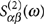is defined in terms of the noise power as1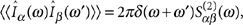where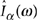, α=L,R, is the Fourier-transformed current and double brackets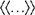denote cumulant averaging over many experimental realizations. Figure 1d shows the Fano factor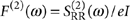for the right tunnelling barrier with the mean current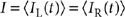being constant in the stationary state. The noise is symmetric in frequency,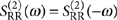, and results are shown for positive frequencies only. For uncorrelated transport, the noise spectrum would be white, that is, F(2)(ω)=1 on all frequencies, corresponding to a Poisson process. Our measurements, in contrast, show a clear suppression of fluctuations below the Poisson value at low frequencies. This is due to the strong Coulomb interactions on the QD that introduce correlations in the stream of electrons propagating through the SET: each electron spends a finite time on the QD during which it blocks the next electron entering the QD. The measured noise spectrum has a Lorentzian shape whose width is determined by the inverse dynamical timescale of the correlations. Our measurements of the finite-frequency noise thereby enable a direct observation of the correlation time τc55 μs of the transport (Fig. 1d).

To corroborate our experimental results, we calculate the noise spectrum of the schematic model in the inset of Figure 1d. Single electrons tunnel from the left electrode onto the QD at rate ΓL and leave it through the right electrode at rate ΓR. The Fano factor is then1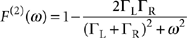where theoretically τc=(ΓLR)−1 is identified as the correlation time. This expression qualitatively explains the measured noise spectrum. Quantitative agreement is obtained by also taking into account the finite detection rate ΓD of the QPC charge-sensing scheme13,37. The three parameters ΓL, ΓR, and ΓD can be independently extracted from the distribution of waiting times between detected tunnelling events14,38,39. The model calculations (see Methods) are in excellent agreement with measurements over the full range of frequencies, demonstrating the high quality of our experimental data.

### Cross-correlations

Cross-correlations between the left IL(t) and the right IR(t) currents can also be measured (Fig. 1d). For the schematic model, the (cross-correlation) Fano factor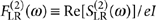reads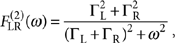which in the zero-frequency limit coincides with the noise spectrum,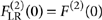, as a consequence of charge conservation on the QD. The two currents are clearly correlated at frequencies that are lower than the inverse correlation time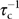, but the cross-correlator eventually reaches zero at higher frequencies. Interestingly, the cross-correlations of the detected pulse currents are slightly negative at high frequencies. This is due to the finite resolution of the QPC charge-sensing protocol that is not able to distinguish current pulses that are separated in time by an interval that is shorter than the inverse detector rate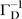.

### Higher order cumulants

We now turn to measurements of higher order finite-frequency cumulants. The mth finite-frequency current correlator corresponding to a time-dependent current I(t) is defined as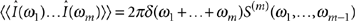where translational invariance in time implies frequency conservation as indicated by the Dirac delta function δ(ω) and S(m)(ω1,...,ωm−1) is the polyspectrum40,41. In the case m=2, the polyspectrum yields the noise power spectrum S(2)(ω), whereas the skewness (or bispectrum) is given by m=3 with the corresponding Fano factor F(3)(ω1,ω2)=S(3)(ω1,ω2)/e2I. We focus here on the frequency-dependent skewness S(3)(ω1,ω2), although our experimental data in principle allows us also to extract cumulants of even higher orders.

The measured skewness (Fig. 2a), shows a much richer structure and frequency dependence compared with the noise spectrum. The skewness obeys several symmetries following from the definition (Fig. 2b). We exploit the mirror symmetry with respect to interchange of frequencies, S(3)(ω1,ω2)=S(3)(ω2,ω1), to compare measurement and model calculations: experimental results are presented above the diagonal (ω1=ω2), while model calculations are shown below (Fig. 2a). The nonzero bispectrum indicates non-Gaussian statistics on all frequencies and shows strong correlations between different spectral components of the current. Importantly, these correlations are not a consequence of non-linearities in the detection scheme, but are solely due to the physical non-equilibrium conditions imposed by the applied voltage bias. The pulse currents are directly derived from the tunnelling events, and the influence of external noise sources, including the amplification of the QPC current, is thereby explicitly avoided. Intuitively, one would expect the correlations to vanish, if the observation frequency is larger than the average frequency of the transport. Surprisingly, however, a certain degree of correlation persists even if one frequency is large, while the other is kept finite. This is in stark contrast to the second Fano factor F(2)(ω) that approaches unity in the high-frequency limit (Fig. 1d), corresponding to uncorrelated tunnelling events.

For the schematic model in Figure 1d, the finite-frequency skewness reads32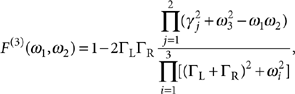having defined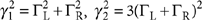, and ω3=ω1+ω2. This expression qualitatively explains the measured finite-frequency skewness and shows that the skewness has a complex structure that is not just a simple Lorentzian-shaped function of frequencies. We note that the third Fano factor F(3)(ω1,ω2) reduces to the zero-frequency limit of the noise F(2)(0) in the limit ω2=0 and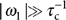. This is immediately visible in Figure 2c. Again, quantitative agreement is achieved by including the finite detection rate ΓD in the model calculations, as illustrated explicitly in Figure 2c–e. The analytic expression shows that the frequency dependence of the skewness, unlike the auto- and cross-correlation noise spectra, is not only governed by the correlation time τc. The skewness has an involved frequency dependence, given by several frequency scales, which cannot be deduced from the noise spectrum alone.

### Higher order cross-correlations

In Figure 3a, we finally consider the cross-bispectrum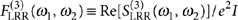, measuring the third-order correlations between tunnelling electrons entering and leaving the SET. The three non-redundant permutations of the left and right pulse currents lead to a reduced symmetry of the cross-bispectrum, as visualized in comparison with the auto-correlation bispectrum in Figure 3a. The cross-bispectrum coincides with the auto-bispectrum on the line ω2=0, where they approach the zero-frequency correlation of the shot noise for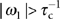(Fig. 3b). In contrast, for |ω2|≠0, the cross-bispectrum shows a frequency dependence similar to the cross-correlation shot noise and the anti-correlations due to the detection process become visible (Fig. 3c).

## Discussion

We have measured the current statistics of charge transport in an SET and directly determined the dynamical features and timescales of the current fluctuations. From the measured frequency-dependent noise power, we extracted the correlation time of the fluctuations. The noise power is a single-frequency quantity only, and to investigate the correlations between different spectral components of the current using bispectral analysis, we measured the frequency-dependent third order correlation function (the skewness). The skewness shows that the current statistics are non-gaussian on all frequencies owing to the applied voltage bias. Our experimental results are supported by model calculations that are in excellent agreement with measurements. The results presented here are important for future applications of SETs in nanoscale electrical circuits operating with single electrons. Our accurate and stable experiment also facilitates several promising directions for basic research on nanoscale quantum devices. These include experimental investigations of fluctuation relations at finite frequencies and higher order noise detection of interaction42 and coherence-induced correlation effects43 in quantum transport under non-equilibrium conditions.

## Methods

### Device fabrication

The device was fabricated by local anodic oxidation techniques using an atomic force microscope on the surface of a GaAs/AlGaAs heterostructure with electron density 4. 6×1015 m−2 and a mobility of 64 m2/Vs. The two-dimensional electron gas residing 34 nm below the heterostructure surface is depleted underneath the oxidized lines, allowing us to define the QD and the QPC.

### Measurements

The experiment was carried out in a 3He cryostat at 500 mK with an applied bias of 900 μV across the QD to ensure unidirectional transport and to avoid the influence of thermal fluctuations. The QPC detector was tuned to the edge of the first conduction step. The current through the QPC was measured with a sampling frequency of 500 kHz. The tunnelling events were extracted from the QPC current using a step detection algorithm and converted into time-dependent pulse currents: Time was discretized in steps of Δt=40 μs and in each step the number of tunnelling events Δn in (out) of the QD was recorded. The current into (out of) the QD at a given time step is then I(t)=(−en/Δt.

#### Error estimates

We estimated the errors of the measured spectra by dividing the experimental data into 30 separate batches. The spectra were determined for each batch individually and their standard deviation was used as a measure of the experimental accuracy.

#### Finite-frequency cumulants

To define the finite-frequency cumulants of the current, we consider the m-time probability distribution32,33 P(m)(n1, t1;...;nm, tm) that nk electrons have been transferred during the time span [0, tk] for k=1,...,m. The corresponding cumulant generating function is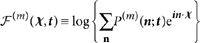with χ=(χ1,...,χm), t=(t1,...,tm), and n=(n1,...,nm). (An equivalent definition uses only a single but time-dependent counting field44.) The m-time cumulants of P(m)(n; t) are then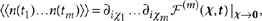with the corresponding m-time cumulants of the current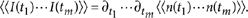These equations define the cumulant averages denoted by double brackets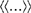. In the Fourier domain, the current cumulants can be expressed as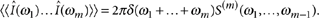as the sum of frequencies is zero in the stationary state. The Fourier-transformed current is denoted as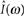and S(m)(ω1, ω2,..., ωm−1) is the polyspectrum40, which for m=2 and m=3 yields the noise spectrum S(2)(ω) (second cumulant) and the bispectrum (third cumulant) S(3)(ω1, ω2), respectively. We note that the noise spectrum S(2)(ω) in the stationary state is a single-frequency quantity that can be related directly to the one-time probability distribution P(1)(n, t) according to MacDonald's formula45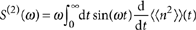The bispectrum F(3)(ω1,ω2) in contrast is a two-frequency quantity that reflects correlations of the current beyond what is captured by P(1)(n, t) alone.

### Theoretical model

We consider the probability vector |p(t)〉=[p00(t), p10(t), p01(t), p11(t)]T, where the first index denotes the number of (extra) electrons on the QD, i=0, 1, and the second index denotes the detected number of (extra) electrons on the QD as inferred from the current through the QPC, j=0, 1. The probability vector evolves according to the rate equation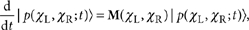having introduced separate counting fields χL and χR that couple to the number of detected electrons that have passed the left and the right barriers, respectively44. The matrix M(χL, χR) reads13,14,37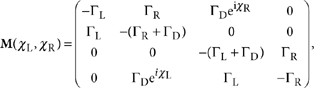where factors of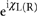in the off-diagonal elements correspond to processes that increase by one the number of detected electrons that have tunnelled across the left (right) barrier (Fig. 1c,d). The detector rate ΓD of the QPC detector scheme tends to infinite for an ideal detector only, but is finite otherwise.

### Calculations

For the calculations of the finite-frequency cumulants it is useful to write the matrix as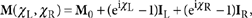where M0=M(0, 0) and IL(R) is the super operator for the detected current through the left (right) barrier46. Additionally, we need the stationary state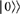, found by solving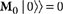and normalized such that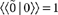, where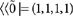. We define the projectors46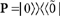and Q=1P and the frequency-dependent pseudoinverse47 R(ω)=Q[iω+M0]−1Q, which is well-defined even in the zero-frequency limit ω→0, since the inversion is performed only in the subspace spanned by Q, where M0 is regular. These objects constitute the essential building blocks for our calculations. The frequency-dependent second and third cumulants, S(2)(ω) and S(3)(ω1,ω2), can then be evaluated following refs 47,48 and 33, respectively.

How to cite this article: Ubbelohde, N. et al. Measurement of finite-frequency current statistics in a single-electron transistor. Nat. Commun. 3:612 doi: 10.1038/ncomms1620 (2012).

## References

1. 1

Blanter, Ya. M. & Büttiker, M. Shot noise in mesoscopic conductors. Phys. Rep. 336, 1 (2000).

2. 2

Nazarov, Yu.V. (ed.) Quantum Noise in Mesoscopic Physics (Kluwer, Dordrecht, 2003).

3. 3

Levitov, L. S. & Lesovik, G. B. Charge-distribution in quantum shot-noise. JETP Lett. 58, 230 (1993).

4. 4

Reulet, B., Senzier, J. & Prober, D. E. Environmental effects in the third moment of voltage fluctuations in a tunnel junction. Phys. Rev. Lett. 91, 196601 (2003).

5. 5

Bylander, J., Duty, T. & Delsing, P. Current measurement by real-time counting of single electrons. Nature 434, 361 (2005).

6. 6

Bomze, Y., Gershon, G., Shovkun, D., Levitov, L. S. & Reznikov, M. Measurement of counting statistics of electron transport in a tunnel junction. Phys. Rev. Lett. 95, 176601 (2005).

7. 7

Gustavsson, S. et al. Counting statistics of single-electron transport in a quantum dot. Phys. Rev. Lett. 96, 076605 (2006).

8. 8

Fujisawa, T., Hayashi, T., Tomita, R. & Hirayama, Y. Bidirectional counting of single electrons. Science 312, 1634 (2006).

9. 9

Sukhorukov, E. V. et al. Conditional statistics of electron transport in interacting nanoscale conductors. Nature Phys. 3, 243 (2007).

10. 10

Fricke, C., Hohls, F., Wegscheider, W. & Haug, R. J. Bimodal counting statistics in single-electron tunneling through a quantum dot. Phys. Rev. B 76, 155307 (2007).

11. 11

Timofeev, A. V., Meschke, M., Peltonen, J. T., Heikkilä, T. T. & Pekola, J. P. Wideband detection of the third moment of shot noise by a hysteretic josephson junction. Phys. Rev. Lett. 98, 207001 (2007).

12. 12

Gershon, G., Bomze, Y., Sukhorukov, E. V. & Reznikov, M. Detection of non-gaussian fluctuations in a quantum point contact. Phys. Rev. Lett. 101, 016803 (2008).

13. 13

Gustavsson, S. et al. Electron counting in quantum dots. Surf. Sci. Rep. 64, 191 (2009).

14. 14

Flindt, C. et al. Universal oscillations in counting statistics. Proc. Natl Acad. Sci. USA 106, 10119 (2009).

15. 15

Zhang, X. C. et al. Tunable electron counting statistics in a quantum dot at thermal equilibrium. Phys. Rev. B 80, 035321 (2009).

16. 16

Gabelli, J. & Reulet, B. Full counting statistics of avalanche transport: An experiment. Phys. Rev. B 80, 161203(R) (2009).

17. 17

Gabelli, J. & Reulet, B. High frequency dynamics and the third cumulant of quantum noise. J. Stat. Mech. P01049 (2009).

18. 18

Xue, W. W. et al. Measurement of quantum noise in a single-electron transistor near the quantum limit. Nature Phys. 5, 660 (2009).

19. 19

Mahé, A. et al. Current correlations of an on-demand single-electron emitter. Phys. Rev. B. 82, 201309(R) (2010).

20. 20

Tobiska, J. & Nazarov, Yu.V. Josephson junctions as threshold detectors for full counting statistics. Phys. Rev. Lett. 93, 106801 (2004).

21. 21

Ankerhold, J. & Grabert, H. How to detect the fourth-order cumulant of electrical noise. Phys. Rev. Lett. 95, 186601 (2005).

22. 22

Zazunov, A., Creux, M., Paladino, E., Creépieux, A. & Martin, T. Detection of finite-frequency current moments with a dissipative resonant circuit. Phys. Rev. Lett. 99, 066601 (2007).

23. 23

Klich, I. & Levitov, L. S. Quantum noise as an entanglement meter. Phys. Rev. Lett. 102, 100502 (2009).

24. 24

Song, H. F., Flindt, C., Rachel, S., Klich, I. & Le Hur, K. Entanglement entropy from charge statistics: exact relations for noninteracting many-body systems. Phys. Rev. B 83, 161408(R) (2011).

25. 25

Förster, H. & Büttiker, M. Fluctuation relations without microreversibility in nonlinear transport. Phys. Rev. Lett. 101, 136805 (2008).

26. 26

Sánchez, R., López, R., Sánchez, D. & Büttiker, M. Mesoscopic Coulomb drag, broken detailed balance, and fluctuation relations. Phys. Rev. Lett. 104, 076801 (2010).

27. 27

Nakamura, S. et al. Nonequilibrium fluctuation relations in a quantum coherent conductor. Phys. Rev. Lett. 104, 080602 (2010).

28. 28

Galaktionov, A. V., Golubev, D. S. & Zaikin, A. D. Statistics of current fluctuations in mesoscopic coherent conductors at nonzero frequencies. Phys. Rev. B 68, 235333 (2003).

29. 29

Nagaev, K. E., Pilgram, S. & Büttiker, M. Frequency scales for current statistics of mesoscopic conductors. Phys. Rev. Lett. 92, 176804 (2004).

30. 30

Pilgram, S., Nagaev, K. E. & Büttiker, M. Frequency-dependent third cumulant of current in diffusive conductors. Phys. Rev. B 70, 045304 (2004).

31. 31

Salo, J., Hekking, F. W. J. & Pekola, J. P. Frequency-dependent current correlation functions from scattering theory. Phys. Rev. B 74, 125427 (2006).

32. 32

Emary, C., Marcos, D., Aguado, R. & Brandes, T. Frequency-dependent counting statistics in interacting nanoscale conductors. Phys. Rev. B 76, 161404(R) (2007).

33. 33

Marcos, D., Emary, C., Aguado, R. & Brandes, T. Finite-frequency counting statistics of electron transport: Markovian theory. New J. Phys. 12, 123009 (2010).

34. 34

Galaktionov, A. V. & Zaikin, A. D. Current fluctuations in composite conductors: beyond the second cumulant. Phys. Rev. B 85, 115418 (2011).

35. 35

Lu, W., Ji, Z. Q., Pfeiffer, L., West, K. W. & Rimberg, A. J. Real-time detection of electron tunnelling in a quantum dot. Nature 423, 422 (2003).

36. 36

Vandersypen, L. M. K. et al. Real-time detection of single-electron tunneling using a quantum point contact. Appl. Phys. Lett. 85, 4394 (2004).

37. 37

Naaman, O. & Aumentado, J. Poisson transition rates from time-domain measurements with a finite bandwidth. Phys. Rev. Lett. 96, 100201 (2006).

38. 38

Brandes, T. Waiting times and noise in single particle transport. Ann. Phys. (Berlin) 17, 477 (2008).

39. 39

Albert, M., Flindt, C. & Bütikker, M. Distributions of waiting times of dynamic single-electron emitters. Phys. Rev. Lett. 107, 086805 (2011).

40. 40

Nikias, C. L. & Mendel, J. M. Signal processing with higher-order spectra. IEEE Signal Proc. Mag. 10, 10 (1993).

41. 41

Kogan, Sh. Electrical Noise and Fluctuations in Solids (Cambridge University Press, 1996).

42. 42

Barthold, P., Hohls, F., Maire, N., Pierz, K. & Haug, R. J. Enhanced shot noise in tunneling through a stack of coupled quantum dots. Phys. Rev. Lett. 96, 246804 (2006).

43. 43

Kießlich, G., Schöll, E., Brandes, T., Hohls, F. & Haug, R. J. Noise enhancement due to quantum coherence in coupled quantum dots. Phys. Rev. Lett. 99, 206602 (2007).

44. 44

Bagrets, D. A. & Nazarov, Yu.V. Full counting statistics of charge transfer in Coulomb blockade systems. Phys. Rev. B 67, 085316 (2003).

45. 45

MacDonald, D. K. C. Spontaneous fluctuations. Rep. Prog. Phys. 12, 56 (1949).

46. 46

Flindt, C., Novotný, T. & Jauho, A.- P. Full counting statistics of nano-electromechanical systems. Europhys. Lett. 69, 475 (2005).

47. 47

Flindt, C., Novotný, T. & Jauho, A.- P. Current noise spectrum of a quantum shuttle. Physica E 29, 411 (2005).

48. 48

Flindt, C., Novotný, T., Braggio, A., Sassetti, M. & Jauho, A.- P. Counting statistics of non-markovian quantum stochastic processes. Phys. Rev. Lett. 100, 150601 (2008).

## Acknowledgements

We thank M. Büttiker, C. Emary, and Yu. V. Nazarov for instructive discussions. W. Wegscheider (Regensburg, Germany) provided the wafer and B. Harke (Hannover, Germany) fabricated the device. The work was supported by BMBF through nanoQUIT (C. Fr., N. U., F. H., and R. J. H.), DFG through QUEST (C. Fr., N. U., F. H., and R. J. H.), the Villum Kann Rasmussen Foundation (C. Fl.), and the Swiss NSF (C. Fl.).

## Author information

All authors conceived the research. N.U., C.Fr. and F.H. carried out the experiment and analysed data. All authors discussed the results. C.Fl. developed theory and performed calculations. N. U., C.Fr., and C.Fl. wrote the manuscript. F.H. and R.J.H. supervised the research. All authors contributed to the editing of the manuscript.

Correspondence to Rolf J. Haug.

## Ethics declarations

### Competing interests

The authors declare no competing financial interests.

## Rights and permissions

Reprints and Permissions

Ubbelohde, N., Fricke, C., Flindt, C. et al. Measurement of finite-frequency current statistics in a single-electron transistor. Nat Commun 3, 612 (2012). https://doi.org/10.1038/ncomms1620

• Accepted:

• Published:

• ### The photon-assisted shot noise of a strongly correlated quantum dot coupled to the Luttinger liquid leads

• Kai-Hua Yang
• , Yong-Xi Dai
• , Xu Wang
• , Huai-Yu Wang
•  & Shao-Shuai Miao

Physica E: Low-dimensional Systems and Nanostructures (2020)

• ### Autonomous conversion of information to work in quantum dots

• Rafael Sánchez
• , Peter Samuelsson
•  & Patrick P. Potts

Physical Review Research (2019)

• ### Fractional charges in conventional sequential electron tunneling

• Roman-Pascal Riwar

Physical Review B (2019)

• ### Modeling and Control of Quantum Measurement-Induced Backaction in Double Quantum Dots

• Wei Cui
•  & Daoyi Dong

IEEE Transactions on Control Systems Technology (2019)

• ### Trispectrum reconstruction of non-Gaussian noise

• Guy Ramon

Physical Review B (2019)